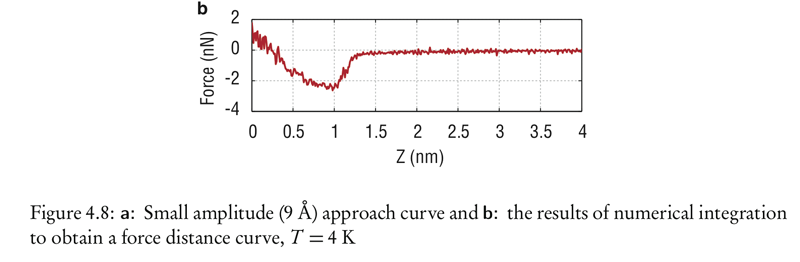A wooden box is released from rest near the top a wooden ramp...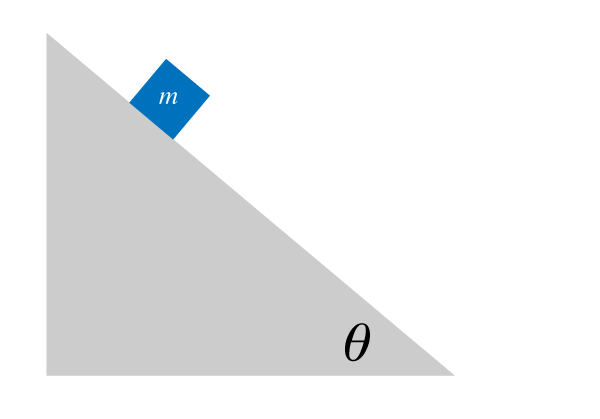# Work and Energy

## Introduction

This is word that means a lot of things depending on the context:

1. Energy Consumption of a Household
2. Energy Drinks
3. Auras & Spiritual Energy
4. Renewable Energy

#### Energy: basicsJames Prescott Joule 1818-1889

Energy is a scalar quantity.

It has SI units of: $\rm kg \cdot m^2/s^2$

The unit is called a Joule, after Mr. Joule

1 Joule = 1 Newton × 1 meter

The different energies of a system can be transferred between each other.## WorkAgain, we might have word problems here.

For us, Work will be defined as the process of transferring energy between a well-defined system and the environment.

The energy may go from the system to the environment. Or, it may go from the environment to the system

#### An example of work

A constant force is applied to this box as it moves across the floor.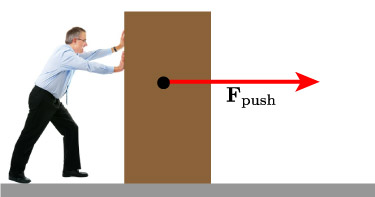The work done is equal given by: $W = F d$.

This is for the case of a constant force which is applied in the same direction as the box is moving.

If Work is equal to Force times Distance, what are the fundamental SI units of Work?

But what if the force is not in the exact same direction.Now we have to consider the component of the force which does point in the direction of the distance traveled.

$$W = F_\parallel d = \mathbf{F} \cdot \mathbf{d} = Fd \cos \theta$$

What is the work being done on the bag by the person carrying it?I swing a ball around my head at constant speed in a circle with circumference 3 m. What is the work done on the ball by the 10 N tension force in the string during one revolution of the ball?

1. 30 J
2. 20 J
3. 10 J
4. 0.333 J
5. 0 J

## Kinetic Energy

We said there we many different forms energy can take (chemical, thermal, potential, etc)

Kinetic Energy is the energy associated with moving objects.

$$\bbox[5px,border:2px solid red]{KE = \frac{1}{2} m v^2}$$

Some example kinetic energies

1. Ant walking: $1 \times 10^{-8}$ J
2. Person walking:$1 \times 10^{-5}$ J
3. Bullet: 5000 J
4. Car @ 100 kph ~ $5 \times 10^5$ J
5. Fast train ~ $1 \times 10^{10}$ J

Two balls of equal size are dropped from the same height from the roof of a building. One ball has twice the mass of the other. When the balls reach the ground, how do the kinetic energies of the two balls compare? (assume no air resistance)

1. The lighter one has one fourth as much kinetic energy as the other does.
2. The lighter one has one half as much kinetic energy as the other does.
3. The lighter one has the same kinetic energy as the other does.
4. The lighter one has twice as much kinetic energy as the other does.
5. The lighter one has four times as much kinetic energy as the other does.

#### Work and Kinetic Energy

Since the work is related to the amount of energy either entering or leaving a system, we can establish a relationship between the work done on an object and the kinetic energy.

$$W = KE_f - KE_0 \ = \frac{1}{2}mv_\textrm{f}^2 - \frac{1}{2}mv_0^2$$

## Potential Energy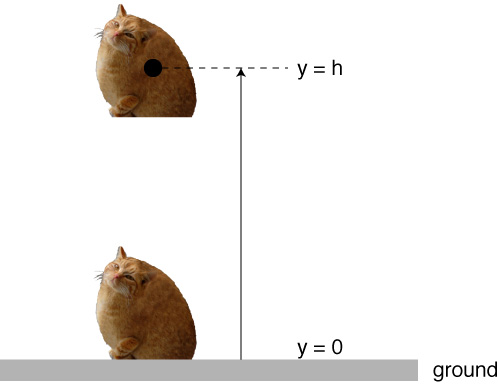We can store energy in a gravitational system by separating the two objects.

The cat, when lifted off the ground, has a gravitational potential energy.

$$\bbox[10px,border:2px solid red]{U_g = mgh}$$

Potential Energy is only dependent on the height!

It doesn’t matter the path an object takes while climbing higher away from the earth. Any route which ends at the same point will impart the same amount of gravitational potential energy to the object.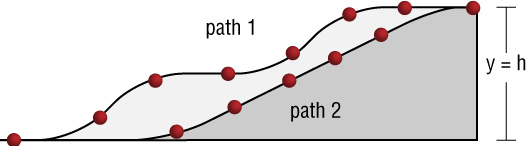#### Work and Potential Energy

Changing the potential energy of an object requires work.

$$\Delta U = -W$$

In the case of gravity:

"The change in the gravitational potential energy of an object is equal to the negative of the work done by the gravitational force"

$$\Delta U_G = -W_G$$

#### Gravity does positive workIn this case we let an object drop, and gravity does positive work.

#### Gravity does negative workIn this case, we raise an object, and gravity does negative work.

#### Work - Energy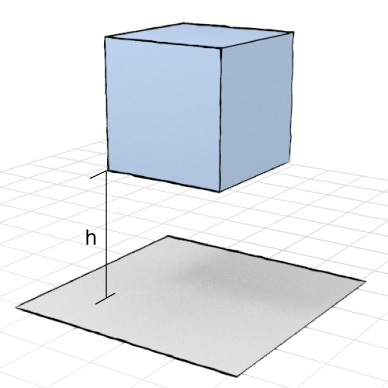Let's now take a look at systems which have both kinetic and potential energy.

For example: this box which is located at a distance $h$ above the ground.

Usually, we'll call the ground $h = 0$.

If these are the only two types of energy in the system, then we have a very special situation.

We can define the total mechanical energy energy of the system as the kinetic + potential.

$$E_\textrm{mechanical} = KE + U$$

## Conservation of Mechanical Energy

If we don't worry about any of those retarding forces like friction or air resistance, then we can say that the total mechanical energy of a system remains the same as time advances.

$$E^\textrm{mech}_i = E^\textrm{mech}_f$$

or

$$\frac{1}{2}mv_i^2 + mgh_i = \frac{1}{2}mv_f^2 + mgh_f$$

Upon dropping a ball, the total mechanical energy is conserved.

$$E_\textrm{mech} = \textrm{constant} = KE + U_\textrm{grav}$$

Knowing this conservation law, we can use either $h$ to find $v$, or $v$ to find $h$.The block starts from rest at point 1. Using the conservation of mechanical energy, determine the speed of the block at point 2.

1. $v = (2gh)^2$
2. $v = \frac{\sqrt{gh}}{2m}$
3. $v = \sqrt{2gh}$
4. $v = 0$The block starts from rest at point 1. Using the conservation of mechanical energy, determine the speed of the block at point 2.

1. $v = (4gh)^2$
2. $v = \frac{\sqrt{gh}}{4m}$
3. $v = \sqrt{2gh}$
4. $v = \sqrt{4gh}$The block starts from rest at point 1. Using the conservation of mechanical energy, determine the speed of the block at point 2.

1. $v = (4gh)^2$
2. $v = \frac{\sqrt{gh}}{4m}$
3. $v = \sqrt{2gh}$
4. $v = \sqrt{4gh}$

#### A car on a hill.

Should this student be worried?

1. Yes
2. No
3. A little bit

A mass $m$ is released from rest on a spherical, frictionless bowl...If $L$ = 4 meters, how high is the ball? That is, find the distance $h$.

#### Block on a rampHere's a 5 kg block on a ramp, where $\theta = 25^\circ$, and h = 20 meters. Find the work done by gravity and the final speed.

## Ex: The Loop the LoopWe saw that if the velocity of the object was large enough, then it would remain inside in contact with the loop.

A successful loop-the-loop looks like this. The ball remains in physical contact with the road at all times. (This is another way of saying the normal force is not zero.)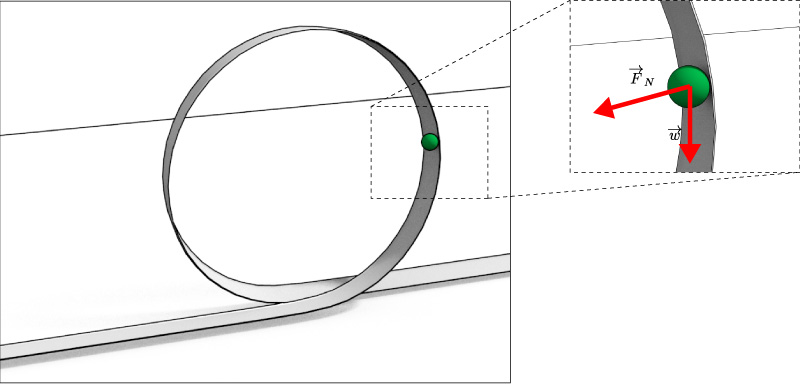An unsuccessful loop-the-loop looks like this. The ball looses physical contact with the road at all times. (This is another way of saying the normal force becomes zero.)

In the case where the velocity is not fast enough to get the ball all the way around the loop, the ball looses contact with the road near the top.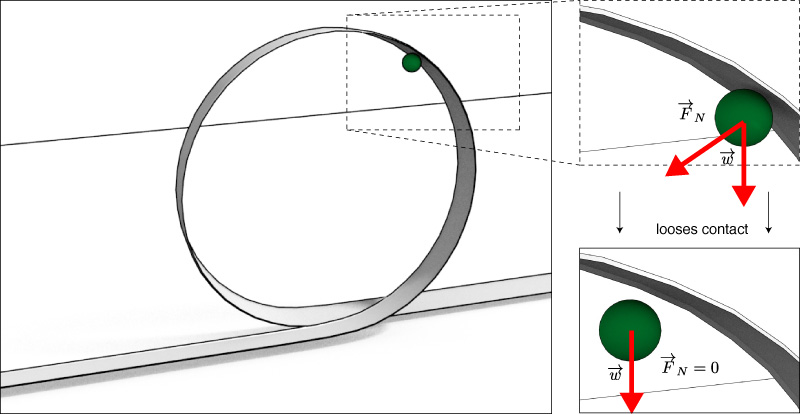At the top of the loop, the sum of forces pointing towards the ground will be given by:

$$\sum{F}_\textrm{ground} = w + F_\textrm{N} = ma$$

Since this is circular motion, we have

$$ma = m \frac{v^2}{r}$$

Thus we can write:

$$\underbrace{mg}_\textrm{weight} + F_N = m\frac{v^2}{r}$$

What is the minimum velocity at the top of the loop needed to complete the loop-the-loop for a regular, no friction, sliding object.

1. $v > \sqrt{rg}$
2. $v > (rg)^2$
3. $v > \sqrt{\frac{r}{g}}$
4. $v > \infty$
5. $v > \sqrt{2rg}$What is an expression for $h$ that would lead to a speed so the ball maintains contact at all times?

## Conservative and Non-conservative Forces

Most of the forces we have seen can be classified as non-conservative.

The exception is Gravity.

Shown are two paths that a box can take to get from point A to point BIf we look at the change in potential energy, $\Delta U_\textrm{grav}$, then we'll see that the Work done by gravity on the box in either case is equal to zero. And, for any other path you can imagine, it's also zero.

$$W = -\Delta U_G = mg(h-h) = 0$$

Moving a box along 2 paths in the presence of friction.The situation is very different if we ask about the work done against friction during these two paths. Path 2 will require more work than path 1.

And so, forces like friction and air resistance are considered non-conservative.

We can however account for these forces in our analysis of certain systems.

$$W_\textrm{NC} = E_f - E_0$$

If the change in energy is non-zero, bewteen the initial and final times of a mechanical system, then there must be some non-conservative forces doing work during the motion.

Now, we have a ramp that has some friction.How can we describe the motion of a box on this ramp?

A child, starting from rest, slides down a slide. On the way down, a kinetic frictional force (a nonconservative force) acts on her. The child has a mass of 50.0 kg, and the height of the slide is 18 m. If the kinetic frictional force does $6.50 \times 10^3$ Joules of work, how fast is the child going at the bottom of the slide?

If the object slides down a hill with an elevation of 10 m, where will it stop? Consider the sloped part frictionless and the rough part at the bottom having a $\mu_k$ equal to 0.3.## Power

Again, here's another word we have to be very careful with. We might be used to calling anything that seems strong 'more powerful'.

For us however, we'll need to restrict our use of the word power to describe how fast a process converts energy.

$$\textrm{Power} = P = \frac{\textrm{change in energy}}{\textrm{time}}$$

The SI unit of power is called the watt: $$\rm 1 watt = 1 joule / second = 1 kg \cdot m^2 / s^3$$

A unit of power in the US Customary system is horsepower:
1 hp = 746 W

Units of [power - time] can also be used to express energy (e.g. kilowatt-hour) $$1 \rm kWh = (1000 W)(3600 s) = 3.6 \times 10^6 J$$We should be able to understand these mysterious documents now.

How many Joules of energy did I use?

How many pushups would I have to do to generate that much energy?

I used to work out on a rowing machine. Each time I pulled on the rowing bar (which simulates the oars), it moved a distance of 1.1 m in a time of 1.6 s. There was a little display that showed my power and it said 90 Watts. How large was the force that I applied to the rowing bar?

A go kart weighs 1000 kg and accelerates at 4 m/s2 for 4 seconds. What is the average power?

## Variable and Constant ForcesIn the most basic situation, the force applied to an object was constant. When this is the case, the Work was given by:

$$W = \mathbf{F} \cdot \mathbf{d}$$

This is also equal to the "area under the curve"

#### Work done by a variable forceHowever, it's also possible that the work will vary as a function of distance. In this case, the work will not be given by the standard form, because, the F is not constant.

$$W \neq \mathbf{F} \cdot \mathbf{d}$$

However, the work is still equal to the "area under the curve"

#### Work done by a variable forceWe don't have the tools (i.e. integration) to be able to do much with this at this level of physics.

#### Work done by a variable force

To deal with these situations, we'll have to use some integration techniques.Here's an applied force that varies as the square of the distance.

We could figure out the area under the curve, which does equal the work, but performing the integral

$$W = \int_{x_i}^{x_f} F(x) dx$$

In this case:

$$W = \int_{x_i}^{x_f} x^2 dx = \frac{1}{3}x^3\biggr|_{x_i}^{x_f}$$

#### In the case of 3d:

If our forces are variable and 3 dimensional, (and perhaps not pointed directly in the direction of the displacement), then we have to use some more developed vector calc:

$$dW = \mathbf{F} \cdot d\mathbf{r} = F_x dx + F_y dy +F_z dz$$

Or, if we integrate over the distance:

$$W = \int_{r_i}^{r_f} dW = \int_{x_i}^{x_f} F_x dx + \int_{y_i}^{y_f} F_y dy + \int_{z_i}^{z_f} F_z dz$$

Since work is a scalar, then these terms will just add up without any complications.

Recover the work-kinetic energy equation?

$$W = \int_{x_i}^{x_f} F(x) dx$$

We should be able to show that this gets back to kinetic energy somehow...

Based on the above:

$$\Delta U(x) = -W = -F \Delta x$$

Now we can take the derivative with respect to x:

$$\bbox[10px ,border:3px solid red]{F(x) = -\frac{dU(x)}{dx}}$$

Based on the the relation between work and potential energy:

$$\Delta U(x) = -W = -F \Delta x$$

Let's rearrange

$$\bbox[10px ,border:3px solid red]{F(x) = -\frac{\Delta U}{\Delta x}}$$

Draw plots of the gravitational potential energy and the gravitational force for an object as a function of height above the earth. Consider only small distances above the surface of the earth.

If the force from an elastic band when stretched is given by $F = -k x$, what would the change in potential energy be for an object attached to the rubber band and pulled back by a distance d? (k is a constant that is determined by the rubber band material ) Plot the force and $U_\textrm{rubber band}$ as functions of distance.

Shown is the potential energy of an unknown force as a function of distance along the x-axis. Where would that force be the largest, and pointed in the positive direction?

A diatomic molecule has two atoms (H2 for example). If the potential energy of such a system is given by:

$$U = \frac{A}{r^{12}} - \frac{B}{r^6}$$

Find the equilibrium separation of such a molecule. That is, find a distance $r$ that will lead to zero net force on each atom.(A and B are just constants)Here are plots of the Potential function, and its derivative (i.e. force).

$$U = \frac{1}{r^{12}}-\frac{1}{r^6}$$

#### Force Microscopy